# NCERT Solutions For Class 11 physics Law of Motion Part 1## myCBSEguide App

CBSE, NCERT, JEE Main, NEET-UG, NDA, Exam Papers, Question Bank, NCERT Solutions, Exemplars, Revision Notes, Free Videos, MCQ Tests & more.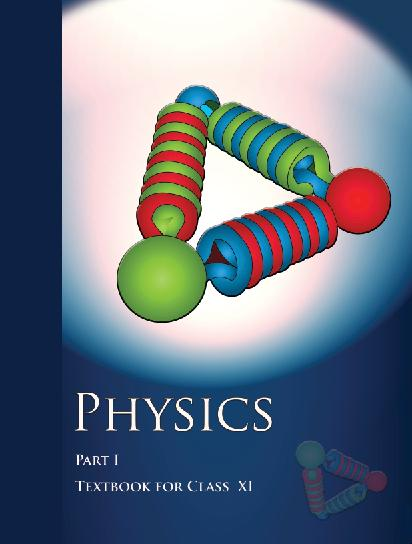## NCERT Class 11 Physics Chapter wise Solutions

• 1 – Physical World
• 2 – Units and Measurements
• 3 – Motion in a Straight line
• 4 – Motion in a Plane
• 5 – Laws of Motion
• 6 – Work, Energy and Power
• 7 – System of Particles and Rotational motion
• 8 – Gravitation
• 9 – Mechanical Properties of Solids
• 10 – Mechanical properties of fluids
• 11 – Thermal Properties of matter
• 12 – Thermodynamics
• 13 – Kinetic Theory
• 14 – Oscillations
• 15 – Waves

### CHAPTER 5 LAWS OF MOTION

• 5.1 Introduction
• 5.2 Aristotle’s fallacy
• 5.3 The law of inertia
• 5.4 Newton’s first law of motion
• 5.5 Newton’s second law of motion
• 5.6 Newton’s third law of motion
• 5.7 Conservation of momentum
• 5.8 Equilibrium of a particle
• 5.9 Common forces in mechanics
• 5.10 Circular motion
• 5.11 Solving problems in mechanic

## NCERT Solutions For Class 11 physics Law of Motion Part 1

1. Give the magnitude and direction of the net force acting on

(a) a drop of rain falling down with a constant speed,

(b) a cork of mass 10 g floating on water,

(c) a kite skillfully held stationary in the sky,

(d) a car moving with a constant velocity of 30 km/h on a rough road,

(e) a high-speed electron in space far from all material objects, and free of electric and magnetic fields.

2. A pebble of mass 0.05 kg is thrown vertically upwards. Give the direction and magnitude of the net force on the pebble,

(a) during its upward motion,

(b) during its downward motion,

(c) at the highest point where it is momentarily at rest. Do your answers change if the pebble was thrown at an angle of 45° with the horizontal direction? Ignore air resistance.

3. Give the magnitude and direction of the net force acting on a stone of mass 0.1 kg,

(a) just after it is dropped from the window of a stationary train,

(b) just after it is dropped from the window of a train running at a constant velocity of 36 km/h,

(c) just after it is dropped from the window of a train accelerating with 1 m s-2,

(d) lying on the floor of a train which is accelerating with 1 m s-2, the stone being at rest relative to the train. Neglect air resistance throughout.

4. One end of a string of length l is connected to a particle of mass m and the other to a small peg on a smooth horizontal table. If the particle moves in a circle with speed v the net force on the particle (directed towards the centre) is:

(i) T, (ii)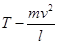, (iii)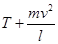, (iv) 0

T is the tension in the string. [Choose the correct alternative].

5. A constant retarding force of 50 N is applied to a body of mass 20 kg moving initially with a speed of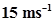. How long does the body take to stop?

6. A constant force acting on a body of mass 3.0 kg changes its speed from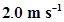to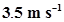in 25 s. The direction of the motion of the body remains unchanged. What is the magnitude and direction of the force?

7. A body of mass 5 kg is acted upon by two perpendicular forces 8 N and 6 N. Give the magnitude and direction of the acceleration of the body.

8. The driver of a three-wheeler moving with a speed of 36 km/h sees a child standing in the middle of the road and brings his vehicle to rest in 4.0 s just in time to save the child. What is the average retarding force on the vehicle? The mass of the three-wheeler is 400 kg and the mass of the driver is 65 kg.

9. A rocket with a lift-off mass 20,000 kg is blasted upwards with an initial acceleration of 5.0 m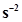. Calculate the initial thrust (force) of the blast.

10. A body of mass 0.40 kg moving initially with a constant speed of 10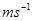to the north is subject to a constant force of 8.0 N directed towards the south for 30 s. Take the instant the force is applied to be t = 0, the position of the body at that time to be x = 0, and predict its position at t= -5 s, 25 s, 100 s.

11. A truck starts from rest and accelerates uniformly at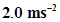. At t = 10 s, a stone is dropped by a person standing on the top of the truck (6 m high from the ground). What are the (a) velocity, and (b) acceleration of the stone at t = 11 s? (Neglect air resistance.)

12. A bob of mass 0.1 kg hung from the ceiling of a room by a string 2 m long is set into oscillation. The speed of the bob at its mean position is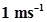. What is the trajectory of the bob if the string is cut when the bob is (a) at one of its extreme positions, (b) at its mean position?

13. A man of mass 70 kg stands on a weighing scale in a lift which is moving

(a) upwards with a uniform speed of 10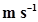,

(b) downwards with a uniform acceleration of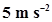,

(c) upwards with a uniform acceleration of.

What would be the readings on the scale in each case?

(d) What would be the reading if the lift mechanism failed and it hurtled down freely under gravity?

14. Figure 5.16 shows the position-time graph of a particle of mass 4 kg. What is the (a) force on the particle for t < 0, t > 4 s, 0 < t < 4 s? (b) impulse at t = 0 and t = 4 s? (Consider one-dimensional motion only).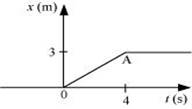Figure 5.16

15. Two bodies of masses 10 kg and 20 kg respectively kept on a smooth, horizontal surface are tied to the ends of a light string. A horizontal force F = 600 N is applied to (i) A, (ii) B along the direction of string. What is the tension in the string in each case?

16. Two masses 8 kg and 12 kg are connected at the two ends of a light inextensible string that goes over a frictionless pulley. Find the acceleration of the masses, and the tension in the string when the masses are released.

17. A nucleus is at rest in the laboratory frame of reference. Show that if it disintegrates into two smaller nuclei the products must move in opposite directions.

18. Two billiard balls each of mass 0.05 kg moving in opposite directions with speed 6 ms-1collide and rebound with the same speed. What is the impulse imparted to each ball due to the other?

19. A shell of mass 0.020 kg is fired by a gun of mass 100 kg. If the muzzle speed of the shell is 80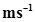, what is the recoil speed of the gun?

20. A batsman deflects a ball by an angle of 45° without changing its initial speed which is equal to 54 km/h. What is the impulse imparted to the ball? (Mass of the ball is 0.15 kg.)

## NCERT Solutions for Class 11 Physics

NCERT Solutions Class 11 Physics PDF (Download) Free from myCBSEguide app and myCBSEguide website. Ncert solution class 11 physics includes text book solutions from both part 1 and part 2. NCERT Solutions for CBSE Class 11 Physics have total 15 chapters. 11 Physics NCERT Solutions in PDF for free Download on our website. Ncert physics class 11 solutions PDF and physics ncert class 11 PDF solutions with latest modifications and as per the latest CBSE syllabus are only available in myCBSEguide

## CBSE app for Class 11

To download NCERT Solutions for class 11 Physics, Chemistry, Biology, History, Political Science, Economics, Geography, Computer Science, Home Science, Accountancy, Business Studies and Home Science; do check myCBSEguide app or website. myCBSEguide provides sample papers with solution, test papers for chapter-wise practice, NCERT solutions, NCERT Exemplar solutions, quick revision notes for ready reference, CBSE guess papers and CBSE important question papers. Sample Paper all are made available through the best app for CBSE students and myCBSEguide website.